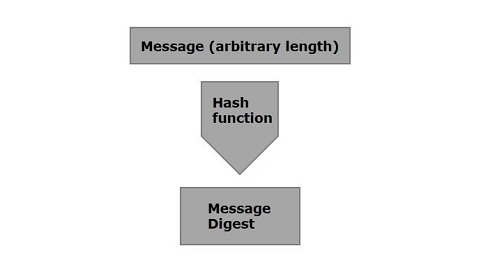# Java Cryptography - Message Digest

Hash functions are extremely useful and appear in almost all information security applications.

A hash function is a mathematical function that converts a numerical input value into another compressed numerical value. The input to the hash function is of arbitrary length but output is always of fixed length.

Values returned by a hash function are called message digest or simply hash values. The following picture illustrated hash function.Java provides a class named MessageDigest which belongs to the package java.security. This class supports algorithms such as SHA-1, SHA 256, MD5 algorithms to convert an arbitrary length message to a message digest.

To convert a given message to a message digest, follow the steps given below −

## Step 1: Create a MessageDigest object

The MessageDigest class provides a method named getInstance(). This method accepts a String variable specifying the name of the algorithm to be used and returns a MessageDigest object implementing the specified algorithm.

Create MessageDigest object using the getInstance() method as shown below.

```MessageDigest md = MessageDigest.getInstance("SHA-256");
```

## Step 2: Pass data to the created MessageDigest object

After creating the message digest object, you need to pass the message/data to it. You can do so using the update() method of the MessageDigest class, this method accepts a byte array representing the message and adds/passes it to the above created MessageDigest object.

```md.update(msg.getBytes());
```

## Step 3: Generate the message digest

You can generate the message digest using the digest() method od the MessageDigest class this method computes the hash function on the current object and returns the message digest in the form of byte array.

Generate the message digest using the digest method.

```byte[] digest = md.digest();
```

### Example

Following is an example which reads data from a file and generate a message digest and prints it.

```import java.security.MessageDigest;
import java.util.Scanner;

public class MessageDigestExample {
public static void main(String args[]) throws Exception{
Scanner sc = new Scanner(System.in);
System.out.println("Enter the message");
String message = sc.nextLine();

//Creating the MessageDigest object
MessageDigest md = MessageDigest.getInstance("SHA-256");

//Passing data to the created MessageDigest Object
md.update(message.getBytes());

//Compute the message digest
byte[] digest = md.digest();
System.out.println(digest);

//Converting the byte array in to HexString format
StringBuffer hexString = new StringBuffer();

for (int i = 0;i<digest.length;i++) {
hexString.append(Integer.toHexString(0xFF & digest[i]));
}
System.out.println("Hex format : " + hexString.toString());
}
}
```

### Output

The above program generates the following output −

```Enter the message
Hello how are you
[B@55f96302
Hex format: 2953d33828c395aebe8225236ba4e23fa75e6f13bd881b9056a3295cbd64d3
```